# RS Aggarwal Solutions for Class 7 Maths Exercise 11A

RS Aggarwal Solutions for Exercise 11A of Class 7 Maths Chapter 11 Profit and Loss, this exercise deals with cost price, selling price. Profit and loss are based on the cost price and selling price of the product. The price at which a product is purchased is called cost price. The price at which an article is sold is called the selling price. Our expert teachers have prepared the RS Aggarwal Solutions for Class 7 Maths Chapter 11 Profit and loss.

## Download the PDF of RS Aggarwal Solutions For Class 7 Maths Chapter 11 Profit and Loss – Exercise 11A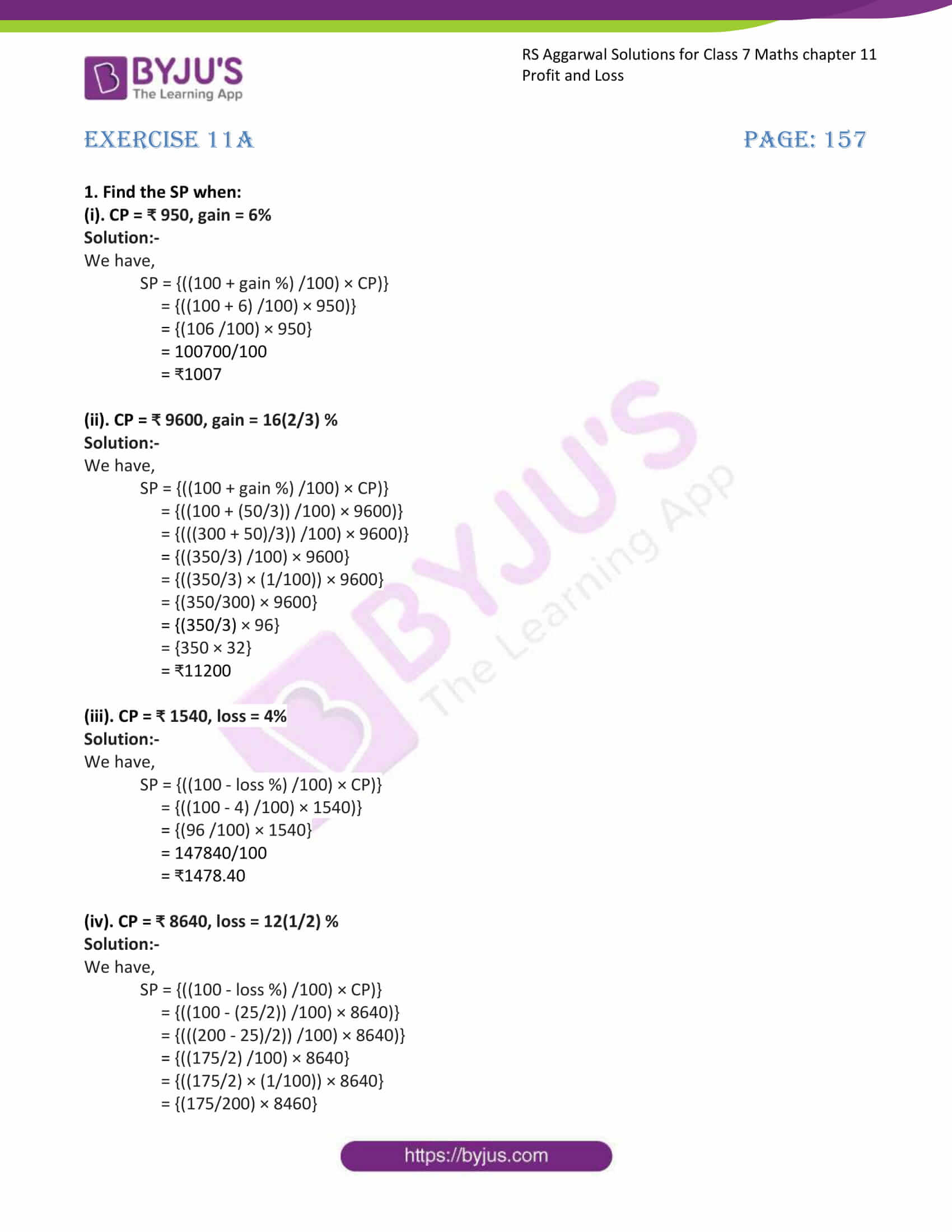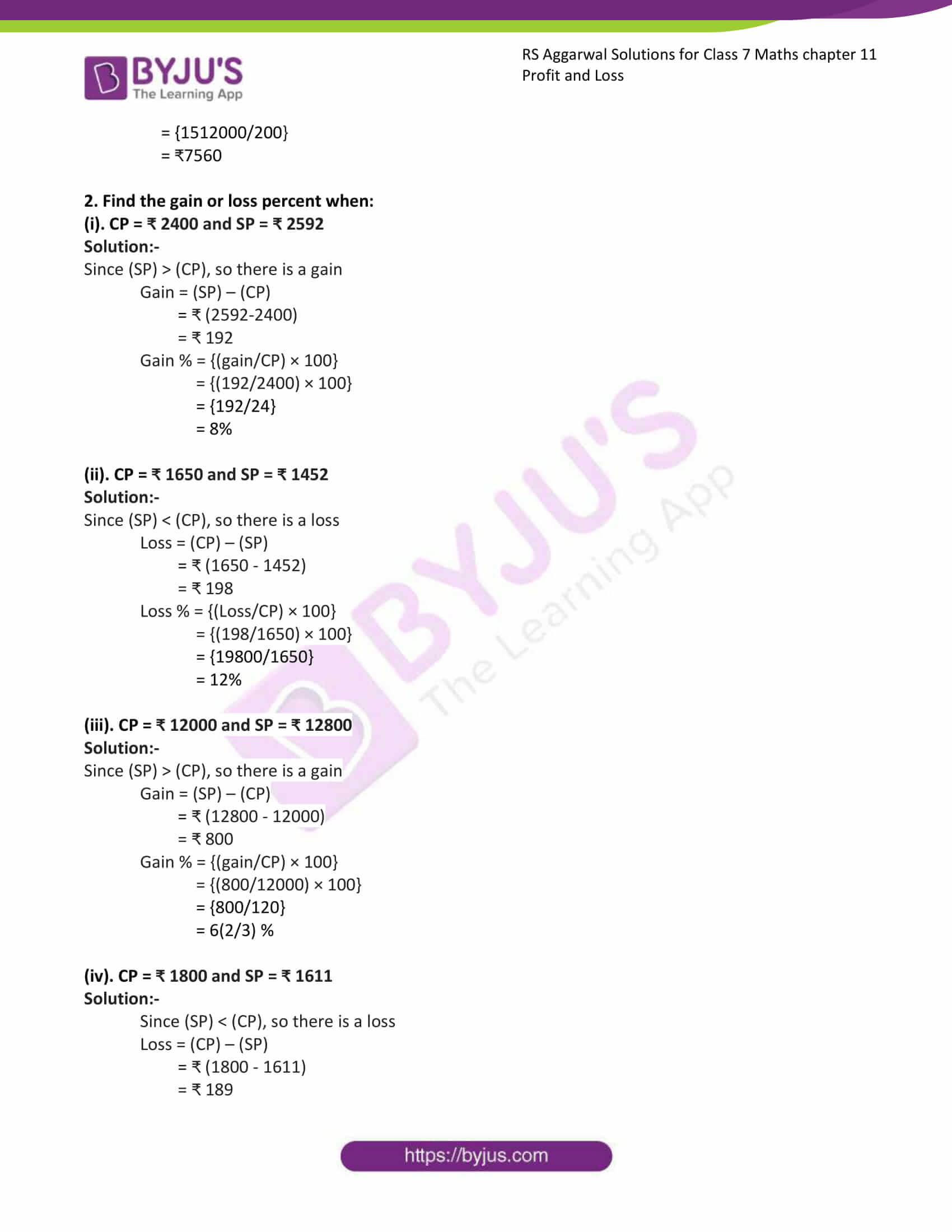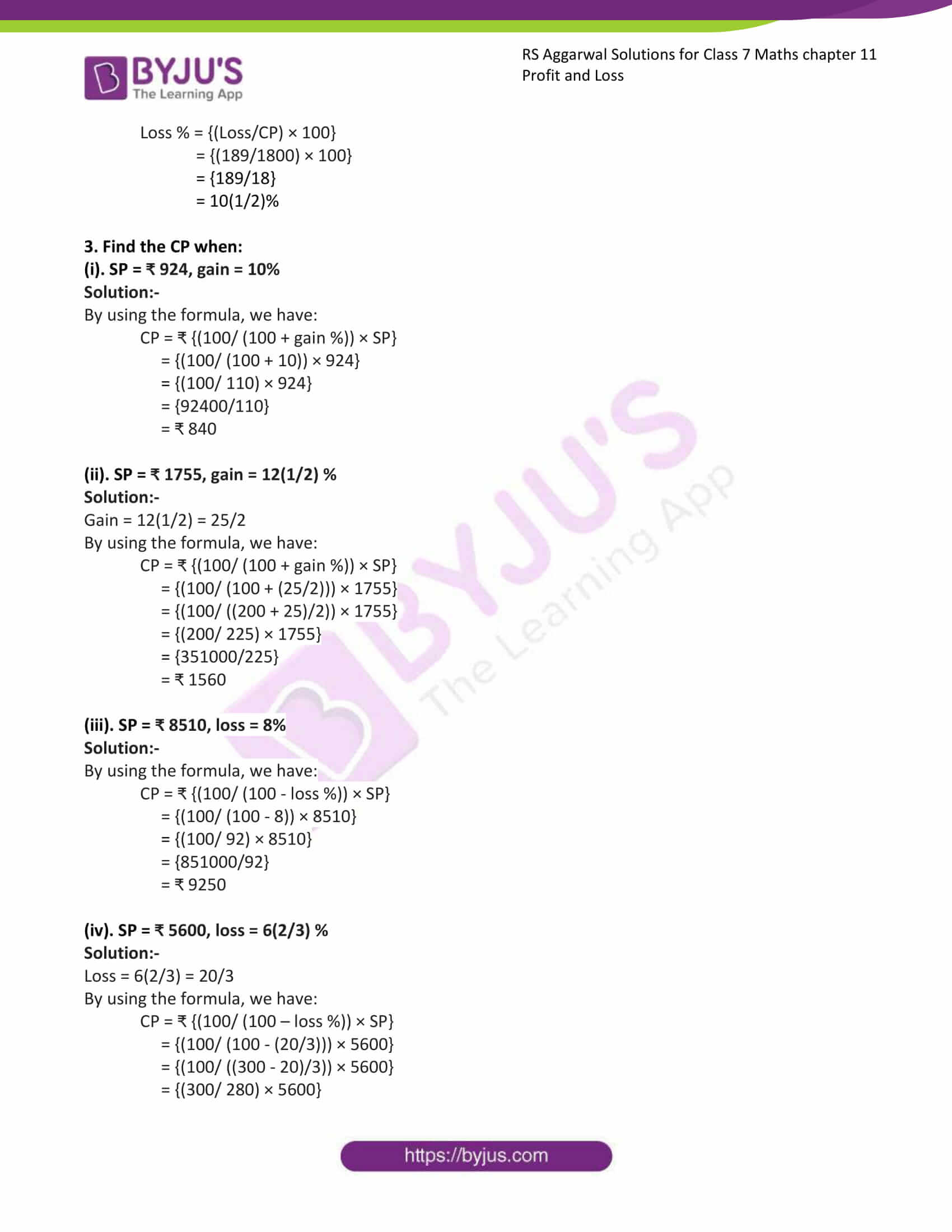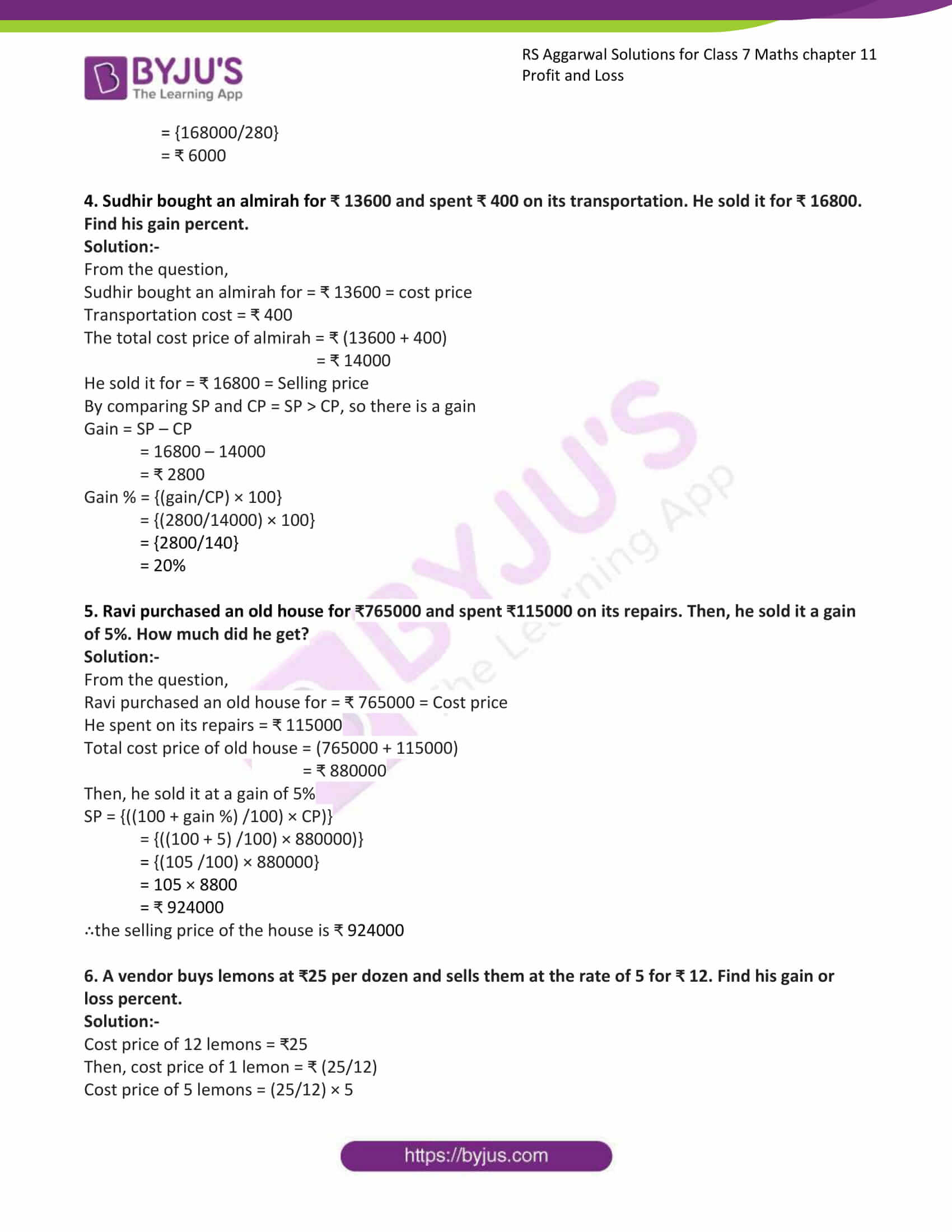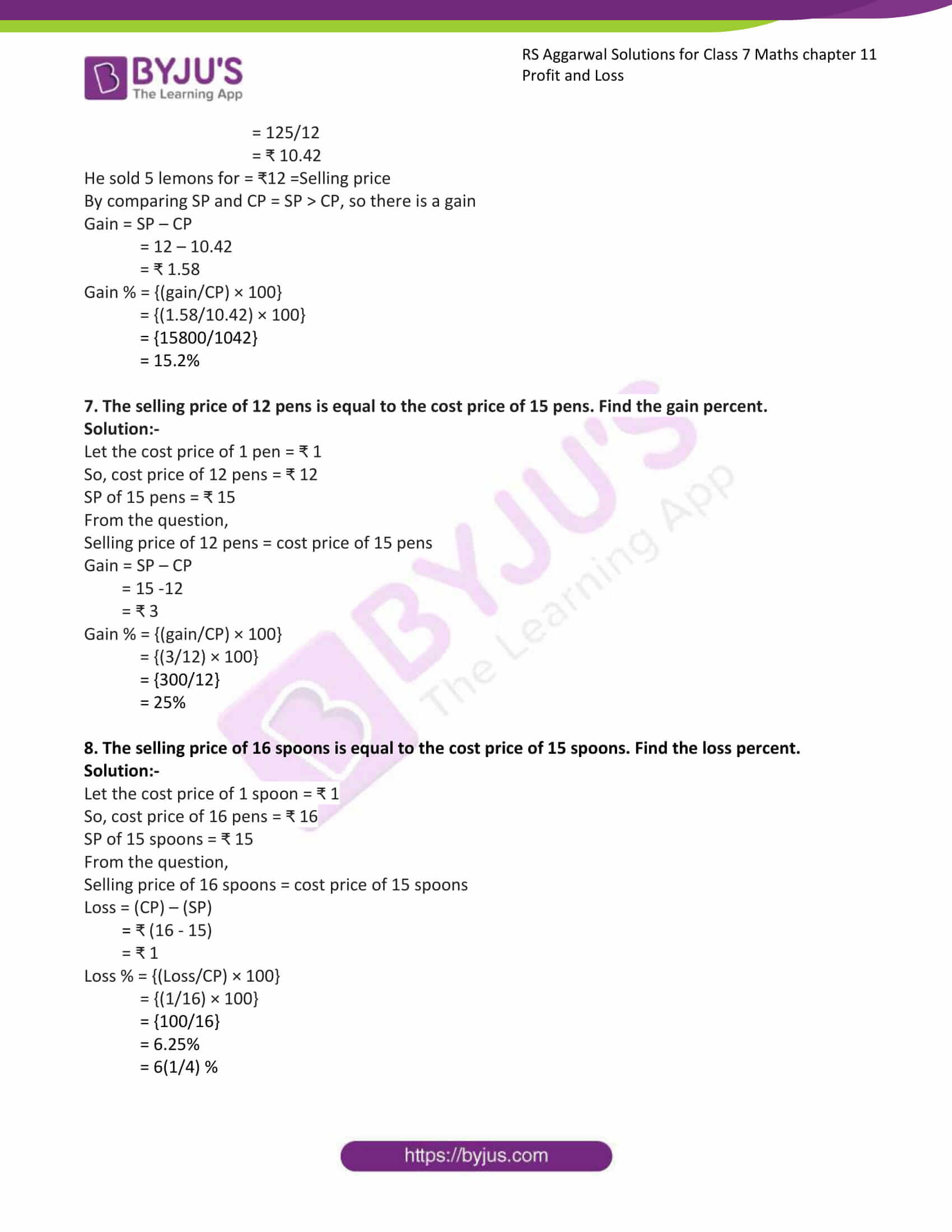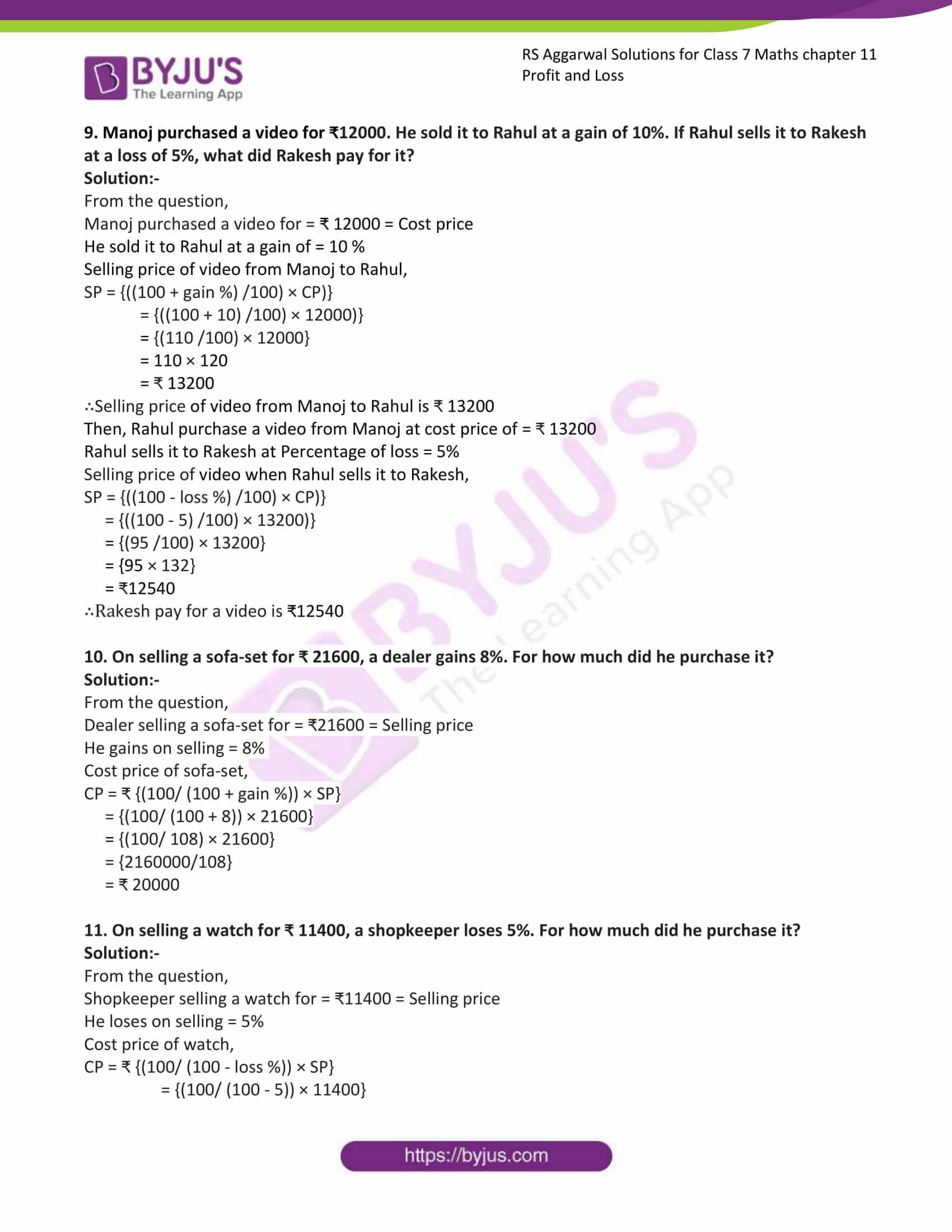### Access answers to Maths RS Aggarwal Solutions for Class 7 Chapter 11 – Profit and Loss Exercise 11A

1. Find the SP when:

(i). CP = ₹ 950, gain = 6%

Solution:-

We have,

SP = {((100 + gain %) /100) × CP)}

= {((100 + 6) /100) × 950)}

= {(106 /100) × 950}

= 100700/100

= ₹1007

(ii). CP = ₹ 9600, gain = 16(2/3) %

Solution:-

We have,

SP = {((100 + gain %) /100) × CP)}

= {((100 + (50/3)) /100) × 9600)}

= {(((300 + 50)/3)) /100) × 9600)}

= {((350/3) /100) × 9600}

= {((350/3) × (1/100)) × 9600}

= {(350/300) × 9600}

= {(350/3) × 96}

= {350 × 32}

= ₹11200

(iii). CP = ₹ 1540, loss = 4%

Solution:-

We have,

SP = {((100 – loss %) /100) × CP)}

= {((100 – 4) /100) × 1540)}

= {(96 /100) × 1540}

= 147840/100

= ₹1478.40

(iv). CP = ₹ 8640, loss = 12(1/2) %

Solution:-

We have,

SP = {((100 – loss %) /100) × CP)}

= {((100 – (25/2)) /100) × 8640)}

= {(((200 – 25)/2)) /100) × 8640)}

= {((175/2) /100) × 8640}

= {((175/2) × (1/100)) × 8640}

= {(175/200) × 8460}

= {1512000/200}

= ₹7560

2. Find the gain or loss percent when:

(i). CP = ₹ 2400 and SP = ₹ 2592

Solution:-

Since (SP) > (CP), so there is a gain

Gain = (SP) – (CP)

= ₹ (2592-2400)

= ₹ 192

Gain % = {(gain/CP) × 100}

= {(192/2400) × 100}

= {192/24}

= 8%

(ii). CP = ₹ 1650 and SP = ₹ 1452

Solution:-

Since (SP) < (CP), so there is a loss

Loss = (CP) – (SP)

= ₹ (1650 – 1452)

= ₹ 198

Loss % = {(Loss/CP) × 100}

= {(198/1650) × 100}

= {19800/1650}

= 12%

(iii). CP = ₹ 12000 and SP = ₹ 12800

Solution:-

Since (SP) > (CP), so there is a gain

Gain = (SP) – (CP)

= ₹ (12800 – 12000)

= ₹ 800

Gain % = {(gain/CP) × 100}

= {(800/12000) × 100}

= {800/120}

= 6(2/3) %

(iv). CP = ₹ 1800 and SP = ₹ 1611

Solution:-

Since (SP) < (CP), so there is a loss

Loss = (CP) – (SP)

= ₹ (1800 – 1611)

= ₹ 189

Loss % = {(Loss/CP) × 100}

= {(189/1800) × 100}

= {189/18}

= 10(1/2)%

3. Find the CP when:

(i). SP = ₹ 924, gain = 10%

Solution:-

By using the formula, we have:

CP = ₹ {(100/ (100 + gain %)) × SP}

= {(100/ (100 + 10)) × 924}

= {(100/ 110) × 924}

= {92400/110}

= ₹ 840

(ii). SP = ₹ 1755, gain = 12(1/2) %

Solution:-

Gain = 12(1/2) = 25/2

By using the formula, we have:

CP = ₹ {(100/ (100 + gain %)) × SP}

= {(100/ (100 + (25/2))) × 1755}

= {(100/ ((200 + 25)/2)) × 1755}

= {(200/ 225) × 1755}

= {351000/225}

= ₹ 1560

(iii). SP = ₹ 8510, loss = 8%

Solution:-

By using the formula, we have:

CP = ₹ {(100/ (100 – loss %)) × SP}

= {(100/ (100 – 8)) × 8510}

= {(100/ 92) × 8510}

= {851000/92}

= ₹ 9250

(iv). SP = ₹ 5600, loss = 6(2/3) %

Solution:-

Loss = 6(2/3) = 20/3

By using the formula, we have:

CP = ₹ {(100/ (100 – loss %)) × SP}

= {(100/ (100 – (20/3))) × 5600}

= {(100/ ((300 – 20)/3)) × 5600}

= {(300/ 280) × 5600}

= {168000/280}

= ₹ 6000

4. Sudhir bought an almirah for ₹ 13600 and spent ₹ 400 on its transportation. He sold it for ₹ 16800. Find his gain percent.

Solution:-

From the question,

Sudhir bought an almirah for = ₹ 13600 = cost price

Transportation cost = ₹ 400

The total cost price of almirah = ₹ (13600 + 400)

= ₹ 14000

He sold it for = ₹ 16800 = Selling price

By comparing SP and CP = SP > CP, so there is a gain

Gain = SP – CP

= 16800 – 14000

= ₹ 2800

Gain % = {(gain/CP) × 100}

= {(2800/14000) × 100}

= {2800/140}

= 20%

5. Ravi purchased an old house for ₹765000 and spent ₹115000 on its repairs. Then, he sold it a gain of 5%. How much did he get?

Solution:-

From the question,

Ravi purchased an old house for = ₹ 765000 = Cost price

He spent on its repairs = ₹ 115000

Total cost price of old house = (765000 + 115000)

= ₹ 880000

Then, he sold it at a gain of 5%

SP = {((100 + gain %) /100) × CP)}

= {((100 + 5) /100) × 880000)}

= {(105 /100) × 880000}

= 105 × 8800

= ₹ 924000

∴the selling price of the house is ₹ 924000

6. A vendor buys lemons at ₹25 per dozen and sells them at the rate of 5 for ₹ 12. Find his gain or loss percent.

Solution:-

Cost price of 12 lemons = ₹25

Then, cost price of 1 lemon = ₹ (25/12)

Cost price of 5 lemons = (25/12) × 5

= 125/12

= ₹ 10.42

He sold 5 lemons for = ₹12 =Selling price

By comparing SP and CP = SP > CP, so there is a gain

Gain = SP – CP

= 12 – 10.42

= ₹ 1.58

Gain % = {(gain/CP) × 100}

= {(1.58/10.42) × 100}

= {15800/1042}

= 15.2%

7. The selling price of 12 pens is equal to the cost price of 15 pens. Find the gain percent.

Solution:-

Let the cost price of 1 pen = ₹ 1

So, cost price of 12 pens = ₹ 12

SP of 15 pens = ₹ 15

From the question,

Selling price of 12 pens = cost price of 15 pens

Gain = SP – CP

= 15 -12

= ₹ 3

Gain % = {(gain/CP) × 100}

= {(3/12) × 100}

= {300/12}

= 25%

8. The selling price of 16 spoons is equal to the cost price of 15 spoons. Find the loss percent.

Solution:-

Let the cost price of 1 spoon = ₹ 1

So, cost price of 16 pens = ₹ 16

SP of 15 spoons = ₹ 15

From the question,

Selling price of 16 spoons = cost price of 15 spoons

Loss = (CP) – (SP)

= ₹ (16 – 15)

= ₹ 1

Loss % = {(Loss/CP) × 100}

= {(1/16) × 100}

= {100/16}

= 6.25%

= 6(1/4) %

9. Manoj purchased a video for ₹12000. He sold it to Rahul at a gain of 10%. If Rahul sells it to Rakesh at a loss of 5%, what did Rakesh pay for it?

Solution:-

From the question,

Manoj purchased a video for = ₹ 12000 = Cost price

He sold it to Rahul at a gain of = 10 %

Selling price of video from Manoj to Rahul,

SP = {((100 + gain %) /100) × CP)}

= {((100 + 10) /100) × 12000)}

= {(110 /100) × 12000}

= 110 × 120

= ₹ 13200

∴Selling price of video from Manoj to Rahul is ₹ 13200

Then, Rahul purchase a video from Manoj at cost price of = ₹ 13200

Rahul sells it to Rakesh at Percentage of loss = 5%

Selling price of video when Rahul sells it to Rakesh,

SP = {((100 – loss %) /100) × CP)}

= {((100 – 5) /100) × 13200)}

= {(95 /100) × 13200}

= {95 × 132}

= ₹12540

∴Rakesh pay for a video is ₹12540

10. On selling a sofa-set for ₹ 21600, a dealer gains 8%. For how much did he purchase it?

Solution:-

From the question,

Dealer selling a sofa-set for = ₹21600 = Selling price

He gains on selling = 8%

Cost price of sofa-set,

CP = ₹ {(100/ (100 + gain %)) × SP}

= {(100/ (100 + 8)) × 21600}

= {(100/ 108) × 21600}

= {2160000/108}

= ₹ 20000

11. On selling a watch for ₹ 11400, a shopkeeper loses 5%. For how much did he purchase it?

Solution:-

From the question,

Shopkeeper selling a watch for = ₹11400 = Selling price

He loses on selling = 5%

Cost price of watch,

CP = ₹ {(100/ (100 – loss %)) × SP}

= {(100/ (100 – 5)) × 11400}

= {(100/ 95) × 11400}

= {11400} × 95

= ₹ 12000

### Access other exercises of RS Aggarwal Solutions For Class 7 Chapter 11 – Profit and Loss

Exercise 11B Solutions